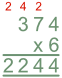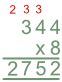Short Multiplication

# Short Multiplication

Basic, GCSE(F),

Multiplication is a value being added to itself a number of times. For example, 6 x 3 takes the number 6 and adds 3 of them together (6 + 6 + 6 = 6 x 3 = 18).

When two numbers are being multiplied and one of them is a single digit, then this is Short Multiplication. Place the numbers in two rows, with the smaller number on the second row. The shorter number is placed in the units column.

Using the smaller number, multiply the larger number one digit at a time, working from right to left. If the result is ten or more, then the tens amount is carried forward and added to the result of the calculation in the next column.

Carry on until all the digits have been multiplied.

## Examples

1. Multiply 374 by 6.Units: 6 x 4 = 24, answer 4 and carry 2 into the tens column
Tens: 6 x 7 = 42, then add the carried 2, = 44, answer 4 and carry 4
Hundreds: 6 x 3 = 18, plus the carried 4, == 22, answer 2 and carry 2
For the thousands, there is only the carried 2 from the previous calculaton.

2. It is 344 miles from Brighton to Newcastle.  A lorry has to complete the return journey four times next week.  How many miles will the lorry have covered altogether on these journeys?